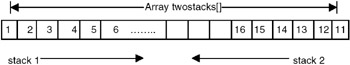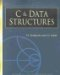# Problems in Stacks and Queues

## PROBLEM CONVERT AN INFIX EXPRESSION TO PREFIX FORM

Program

```#include
#include
#include

#define N 80

typedef enum {FALSE, TRUE} bool;

#include "stack.h"
#include "queue.h"

#define NOPS 7

char operators [] = "()^/*+-";
int priorities[] = {4,4,3,2,2,1,1};
char associates[] = " RLLLL";

char t[N]; char *tptr = t; // this is where prefix will be saved.

int getIndex( char op ) {
/*
* returns index of op in operators.
*/
int i;
for( i=0; i−1;
}

int getPriority( char op ) {
/*
* returns priority of op.
*/
return priorities[ getIndex(op) ];
}

char getAssociativity( char op ) {
/*
* returns associativity of op.
*/
return associates[ getIndex(op) ];
}
void processOp( char op, queue *q, stack *s ) {
/*
* performs processing of op.
*/
switch(op) {
case ')':
printf( "	 S pushing )...
" );
sPush( s, op );
break;
case '(':
while( !qEmpty(q) ) {
*tptr++ = qPop(q);
printf( "	Q popping %c...
", *(tptr-1) );
}
while( !sEmpty(s) ) {
char popop = sPop(s);
printf( "	S popping %c...
", popop );
if( popop == ')' )
break;
*tptr++ = popop;
}
break;
default: {
int priop; // priority of op.
char topop; // operator on stack top.
int pritop; // priority of topop.
char asstop; // associativity of topop.
while( !sEmpty(s) ) {
priop = getPriority(op);
topop = sTop(s);
pritop = getPriority(topop);
asstop = getAssociativity(topop);

if( pritop < priop || (pritop == priop && asstop == 'L') || topop == ')' ) // IMP.
break;
while( !qEmpty(q) ) {
*tptr++ = qPop(q);
printf( "	Q popping %c...
", *(tptr-1) );
}
*tptr++ = sPop(s);
printf( "	S popping %c...
", *(tptr-1) );
}
printf( "	S pushing %c...
", op );
sPush( s, op );
break;
}
}
}

bool isop( char op ) {
/*
* is op an operator?
*/
return (getIndex(op) != −1);
}

char *in2pre( char *str ) {
/*
* returns valid infix expr in str to prefix.
*/
char *sptr;
queue q = {NULL};
stack s = NULL;
char *res = (char *)malloc( N*sizeof(char) );
char *resptr = res;

tptr = t;
for( sptr=str+strlen(str)−1; sptr!=str−1; -sptr ) {
printf( "processing %c tptr-t=%d...
", *sptr, tptr-t );
if( isalpha(*sptr) ) // if operand.
qPush( &q, *sptr );
else if( isop(*sptr) ) // if valid operator.
processOp( *sptr, &q, &s );
else if( isspace(*sptr) ) // if whitespace.
;
else {
fprintf( stderr, "ERROR:invalid char %c.
", *sptr );
return "";

}
}
while( !qEmpty(&q) ) {
*tptr++ = qPop(&q);
printf( "	Q popping %c...
", *(tptr-1) );
}
while( !sEmpty(&s) ) {
*tptr++ = sPop(&s);
printf( "	S popping %c...
", *(tptr-1) );
}
*tptr = 0;
printf( "t=%s.
", t );
for( -tptr; tptr!=t−1; -tptr ) {
*resptr++ = *tptr;
}
*resptr = 0;

return res;
}

int main() {
char s[N];

puts( "enter infix freespaces max 80." );
gets(s);
while(*s) {
puts( in2pre(s) );
gets(s);
}

return 0;
}
```

Explanation

1. In an infix expression, a binary operator separates its operands (a unary operator precedes its operand). In a postfix expression, the operands of an operator precede the operator, while in a prefix expression the operator precedes its operands. Like postfix, a prefix expression is parenthesis-free, that is, any infix expression can be unambiguously written in its prefix equivalent without the need for parentheses.
2. When an infix expression is converted to reverse-prefix, it is scanned from right to left. A queue of operands is maintained, noting that the order of operands in infix and prefix remains the same. Thus, while scanning the infix expression, whenever an operand is encountered, it is pushed in a queue. If the scanned element is a right parenthesis (‘)’), it is pushed in a stack of operators. If the scanned element is a left parenthesis (‘(‘), the queue of operands is emptied to the prefix output followed by popping of all the operators but excluding a right parenthesis in the operator stack.
3. If the scanned element is an arbitrary operator o, then the stack of operators is checked for operators with greater priority than the priority for o. Such operators are popped and written to the prefix output after emptying the operand queue. The operator o is finally pushed in the stack.
4. When the scanning of the infix expression is complete, first the operand queue and then the operator stack are emptied to the prefix output. Any whitespace in the infix input is ignored. Thus the prefix output we get can be reversed to get the required prefix expression of the infix input.
5. Example: If the infix expression is a*b+c/d, then different snapshots of the algorithm while scanning the expression from right to left are as follows:

step

remaining expression

scanned element

queue of operands

stack of operators

prefix output

0

a*b+c/d

nil

empty

empty

nil

1

a*b+c/

d

d

empty

nil

2

a*b+c

/

d

/

nil

3

a*b+

c

d c

/

nil

4

a*b

+

empty

+

dc/

5

a*

b

b

+

dc/

6

a

*

b

* +

dc/

7

nil

a

b a

* +

dc/

8

nil

nil

empty

empty

dc/ba*+

The final prefix output we get is dc/ba*+, whose reverse is +*ab/cd, which is indeed the prefix equivalent of the input infix expression a*b+c*d. Note that all the operands are simply pushed to the queue in steps 1, 3, 5, and 7. In step 2, the operator / is pushed to the empty stack of operators.

In step 4, the operator + is checked against the elements in the stack. Since / (division) has higher priority than + (addition), the queue is emptied to the prefix output (thus we get ‘dc’ as the output) and then the operator / is written (thus we get ‘dc/’ as the output). The operator + is then pushed to the stack. In step 6, the operator * is checked against the stack elements. Since * has a higher priority than +, * is pushed to the stack. Step 8 signifies that all of the infix expression has been scanned. Thus, the queue of operands is emptied to the prefix output (to get ‘dc/ba’), followed by emptying of the stack of operators (to get ‘dc/ba*+’).

Points to Remember

1. A prefix expression is parenthesis-free.
2. When an infix expression is converted to its postfix equivalent, it is scanned from right to left. The prefix expression we get is the reverse of the required prefix equivalent.
3. Conversion of infix to prefix requires a queue of operands and a stack, as in the conversion of an infix to postfix.
4. The order of operands in a prefix expression is the same as that in its infix equivalent.
5. If the scanned operator o1 and the operator o2 at the stack top have the same priority, then the associativity of o2 is checked. If o2 is right associative, it is popped from the stack.

## PROBLEM IMPLEMENTATION OF TWO STACKS USING AN ARRAY

Two stacks are represented in an array twostacks[0…N–1], such that one stack starts from start of the array and grows towards its end while the other one starts from the end of the array and grows towards the start. Thus at any time, the maximum number of elements that the two stacks together can accommodate is N. Write functions Push(stacki, data) and Delete(stacki) to add element data and to delete an element from stack number stacki, 1 <= stacki <= 2. The functions should be able to add elements to the stacks as long as there are fewer than N elements in both stacks together.

Program

#include #define N 10 // combined size of the two stacks. #define EINDEXOUTOFBOUND −1 // error code on overflow in the stacks. #define SUCCESS 0 // success code. typedef int type; // type of each data item. type twostacks[N]; // stacks implemented using array. int stop1 = −1; // pointer for stack 1. int stop2 = N; // pointer for stack 2. int sPush( int stacki, type data ) { /* * pushes data on top of stacki. * returns error on overflow. */ if( stop2-stop1 == 1 ) // overflow. return EINDEXOUTOFBOUND; if( stacki == 1 ) { // first stack. twostacks[ ++stop1 ] = data; } else { // second stack. twostacks[ -stop2 ] = data; } return SUCCESS; } int sDelete( int stacki ) { /* * deletes element at top from stacki. */ printf( "deleting from stack %d... ", stacki ); if( stacki == 1 ) { // first stack. if( stop1 >= 0 ) -stop1; else return EINDEXOUTOFBOUND; } else { // second stack. if( stop2 < N ) ++stop2; else return EINDEXOUTOFBOUND; } return SUCCESS; } void sPrint() { /* * prints the two stacks. */ int i; for( i=0; i<=stop1; ++i ) printf( "%d ", twostacks[i] ); printf( ": " ); for( i=stop2; i

Explanation

1.
2. The two stacks are characterized by their indices (stack pointers). Index 1 starts from −1 and goes up to N − 1 while index 2 starts from N and goes up to 0. When index 1 equals −1, it signifies that stack 1 is empty. When index 2 equals to N, that signifies that stack 2 is empty.
3.
4. If the array is full, indices 1 and 2 point to consecutive elements. This situation is characterized as (index2 − index1 == 1).5.
6. In Push(stacki,data), this condition is checked to signify overflow. If space is available, insertion of the element proceeds. If stacki == 1 then index 1 is incremented and the data is inserted at the index. If stacki == 2, index 2 is decremented and the data is inserted at the index.
7.
8. In Delete(stacki), the condition for underflow is checked. If stacki == 1 then index 1 == −1 signifies underflow of stack 1. If stacki == 2 then index 2 == N signifies underflow of stack 2. If an element exists, then deletion of the element at the stack top proceeds. If stacki == 1 then index 1 is decremented, whereas if stacki == 2 then index 2 is incremented.
9.

Points to Remember

1.
2. To implement two stacks using an array, the two stacks should grow in opposite directions.
3.
4. The maximum number of elements that the two stacks together can accommodate at any time is equal to the size of the array.
5.
6. For an empty stack, making the stack pointer point to an element beyond the first element helps in an insertion, without needing to check for an extra condition. Thus, since we have a stack index/value of −1 during insertion, we need simply to increment it to point to the next empty slot. This is not possible with pointers as we need to explicitly check it for being NULL as in

```if( stacktop == NULL )
error("stack empty.");.
```C & Data Structures (Charles River Media Computer Engineering)
ISBN: 1584503386
EAN: 2147483647
Year: 2006
Pages: 232